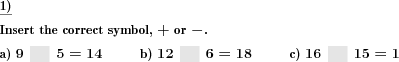Custom math worksheets at your fingertipsDetails for problem "Addition or subtraction, insert correct symbol"

Quickname: 4599

Elementary School, Primary School.

Summary

In a series of addition or subtraction problems, the plus or minus symbol is missing.

ExampleDescription

Enhance and review the skill of addition and subtraction as well as reviewing mathematical symbols by using this type of problem on your worksheet. This is a cloze task where arithmetic signs are missing in simple terms with two operands.

The terms are addition terms and subtraction terms. In each case, the summands and the sum are given for addition tasks or minuend, subtrahend, and difference for subtraction tasks. The arithmetic symbol is missing in each case and has been replaced in the task by a space symbol.

This sort of worksheet can be used as a small or whole group activity or independent/ homework practice additionally. The complexity level can be personalized to use these worksheets from grade 1 to grade 4.

Practicing these problems can help achieve the following array of targets.

1. Revise the concept of addition and subtraction.
2. Understand the relationship between counting to addition and subtraction.
3. Build a strong concept of the equal sign.
4. Use the prediction skills by comparing both sides of the equation.
5. Discover the accuracy of equations of addition and subtraction.

The number of tasks is adjustable. The number of problems can range from 1 to 10. The selection of numbers starts from 10 to 100,000. The correct arithmetic symbol can be derived from the three given operands and is to be inserted accordingly.

The answer key consists of a correct symbol placement.

Download free printable worksheets for this math problem here. The worksheet contains the problems only, the solution sheet includes the answers. Just click on the respective link.

•Worksheet 1Solution sheet with answers
•Worksheet 2Solution sheet with answers
•Worksheet 3Solution sheet with answers

If you can not see the solution sheets for download, they may be filtered out by an ad blocker that you may have installed. If this is the case, please allow ads for this page and reload the page. The solution sheets will then reappear.

• Do these sample worksheets do not really fit?
• Do you need more math worksheets, with a different level of difficulty?
• Would you like to combine different problems on a worksheet and adjust them to your needs?
• As a teacher, you can put together your own worksheets using the automatically generated math problems provided.
With a free initial credit, you can start creating your own math worksheets in a few minutes.

You can try it for free! Register here, to create custom worksheets now!

Customization options for this problem

Parameter
Possible values
Number of problems
1, 2, 3, 4, 5, 6, 7, 8, 9, 10
Number range
10, 20, 30, 40, 50, 100, 200, 500, 1000, 10000, 100000

Similar problems

Remark
Description
Insert correct symbol, no list of possible choices
In an equation with two operands, insert the correct arithmetic symbol.Deutsche Version dieser Aufgabe
These informational pages with samples describe math problems that can be combined on custom math worksheets with solutions for home and K-12 school use.
Deutsche Seiten
×